# [原创]-Day4.数据可视化拓展图形# Seaborn 画图

• 箱线图

• 热力图

• 雷达图

• 二元变量分布

• 成对关系。

## 箱线图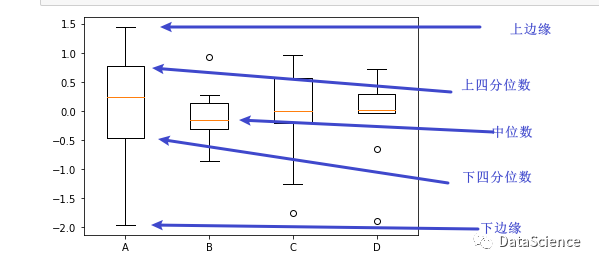# 数据准备
# ⽣成0-1之间的10*4维度数据(10行，4列的数组)
import numpy as np
data = np.random.normal(size=(10,4))
lables = ['A', 'B', 'C', 'D']

# ⽤Matplotlib画箱线图
# boxplot(x,labels=None)函数，x代表绘图数据，labels是缺省值，可以为箱线图添加标签。
import matplotlib.pyplot as plt
plt.boxplot(data, labels=lables) #注意单词labels和lables
plt.show()

# ⽤Seaborn画箱线图
# boxplot(x=None,y=None,data=None)函数。data为DataFrame类型，x、y是data中的变量。
import seaborn as sns
import pandas as pd
df = pd.DataFrame(data, columns=lables)
sns.boxplot(data=df)
plt.show()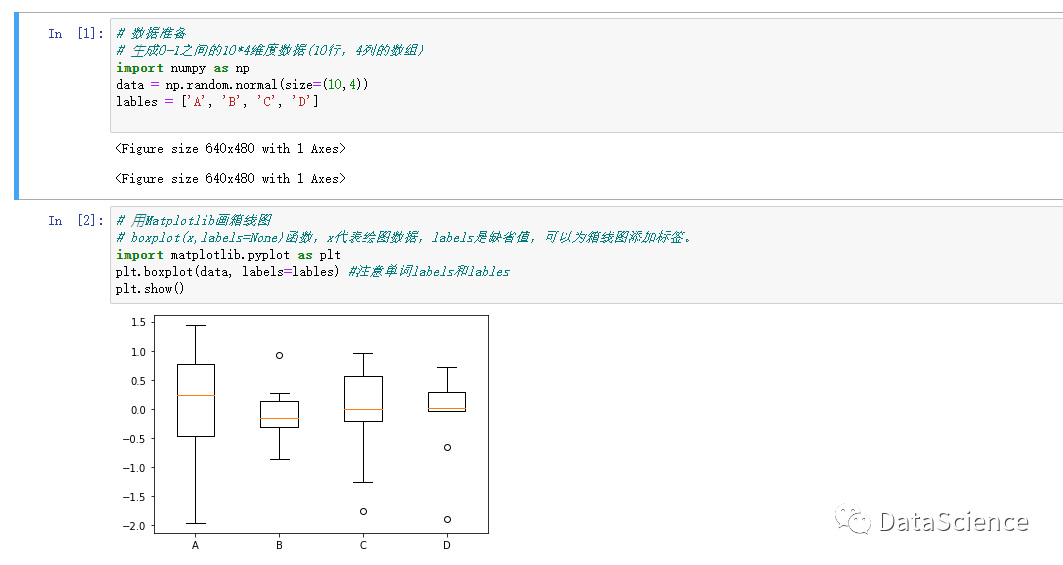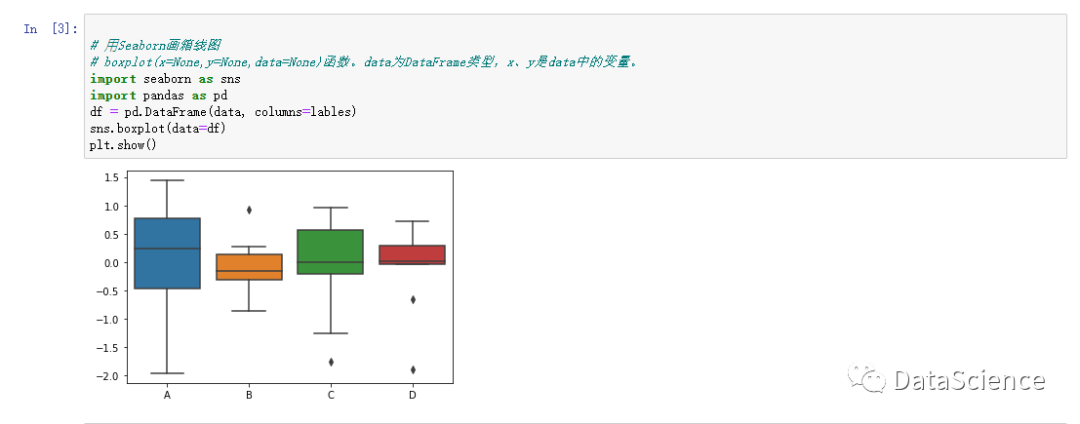## 热力图

### 1、基本热力图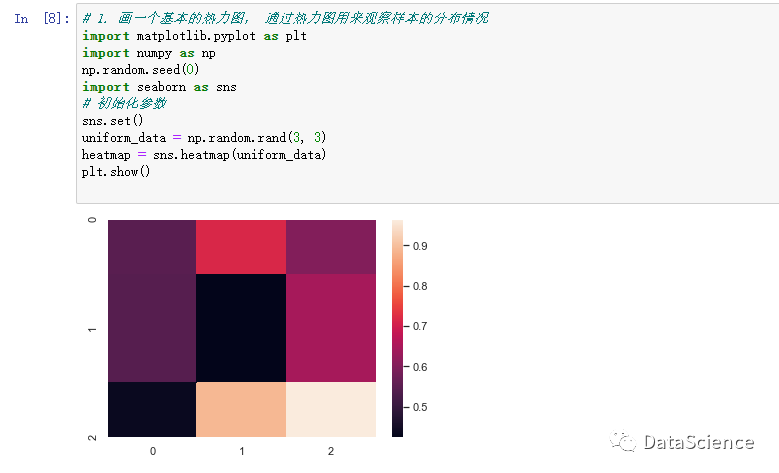### 2、设置热力图区间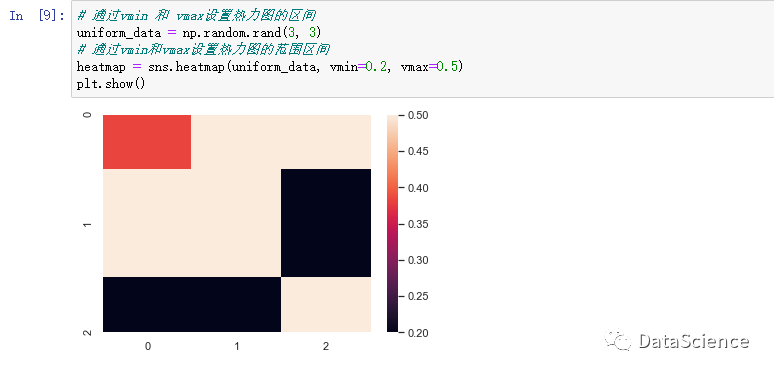### 3、颜色差异更大的原因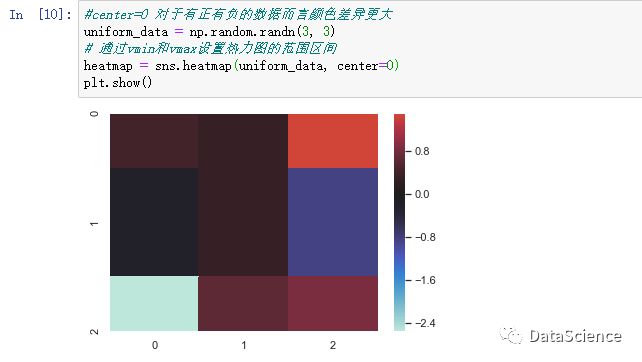### 4、使用Seaborn自带数据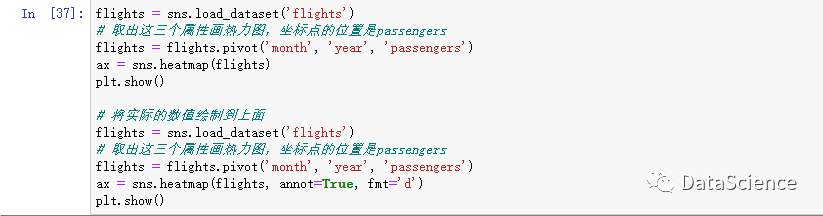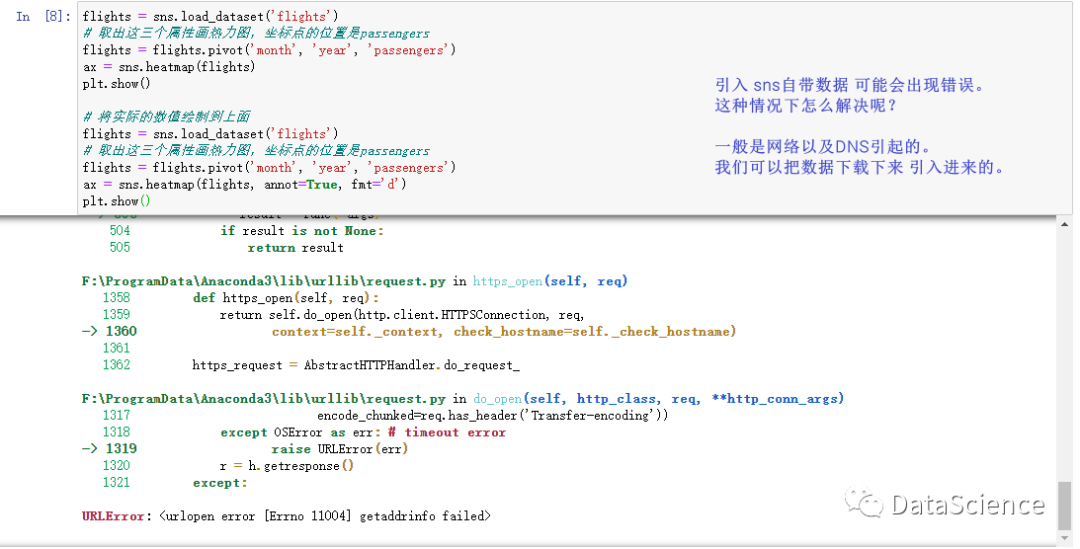下载地址：https://github.com/mwaskom/seaborn-data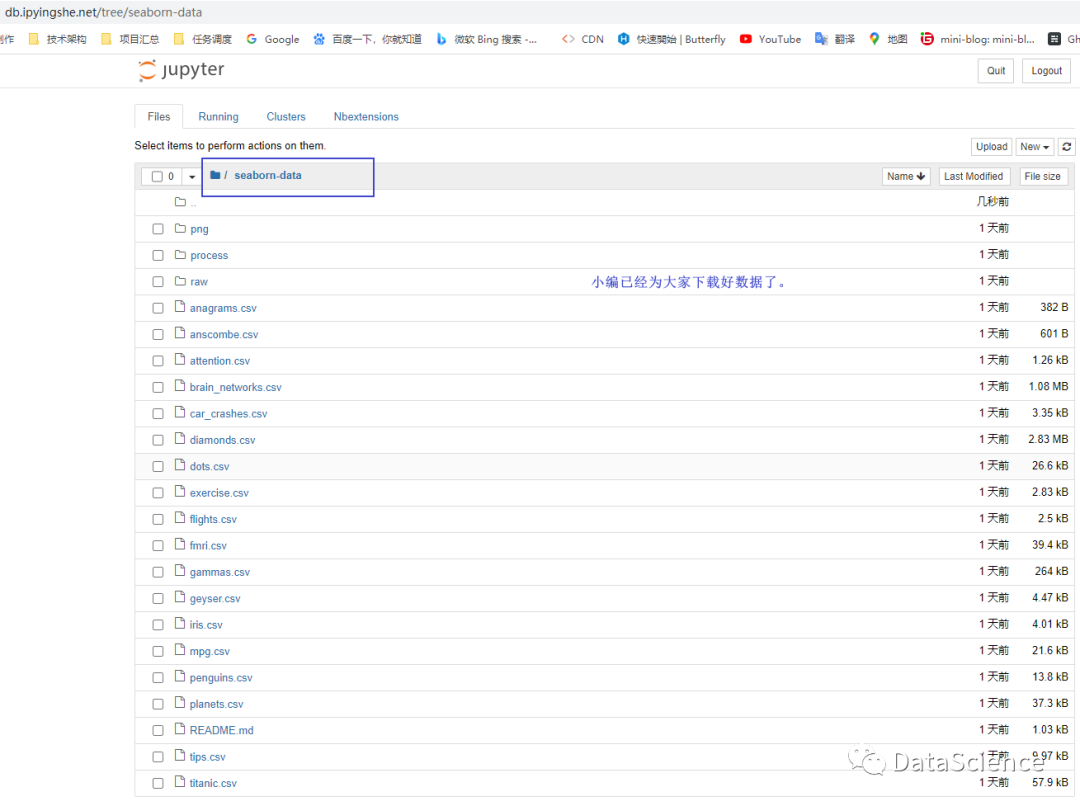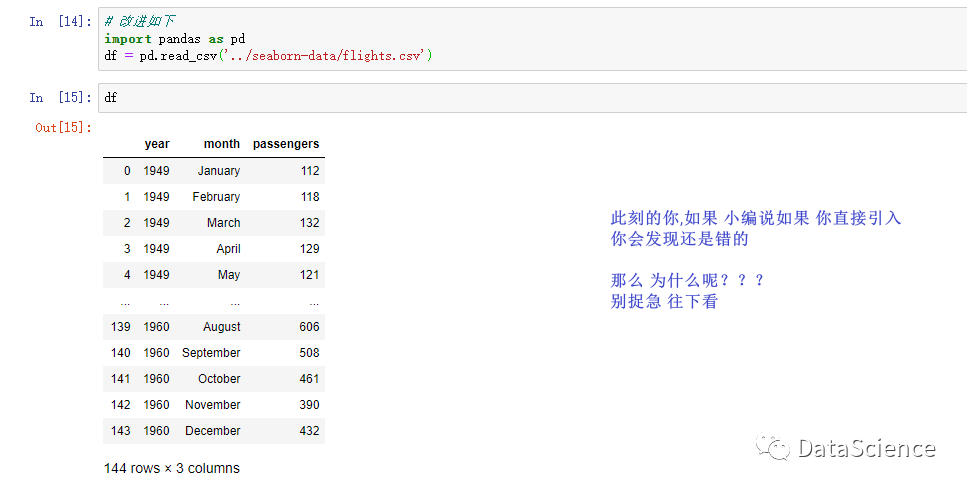数据透视表

• 直接读入进来还是不可的 需要对表进行重塑

• 转化为数据透视表才可以使用  hhh  蒙蔽了吧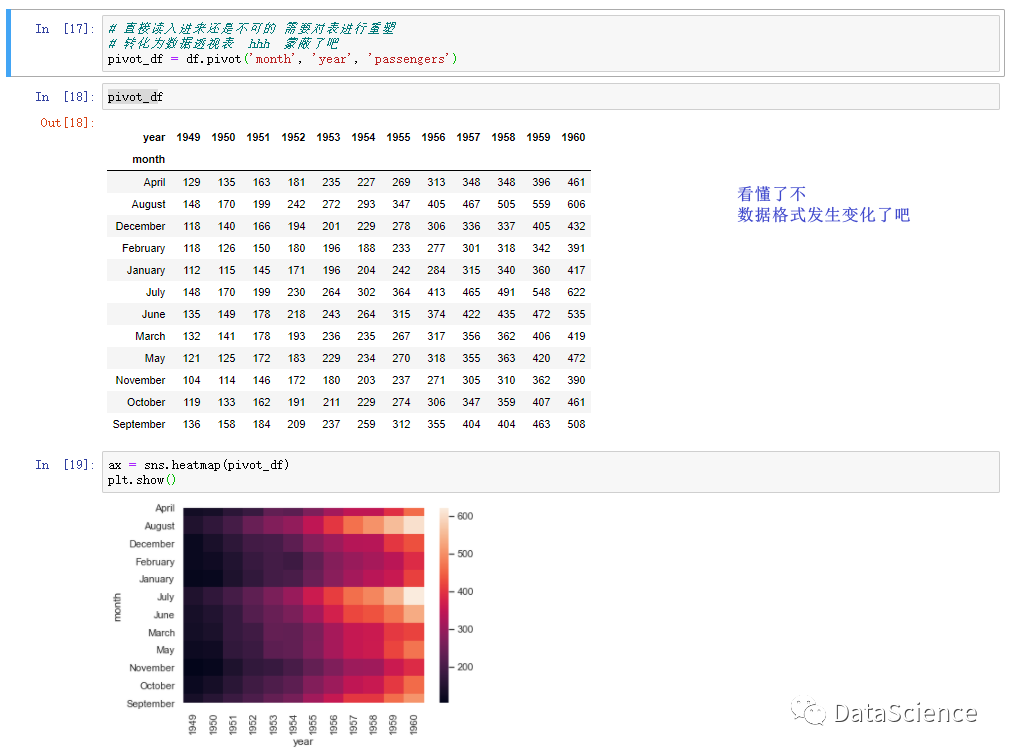### 5、指定调色板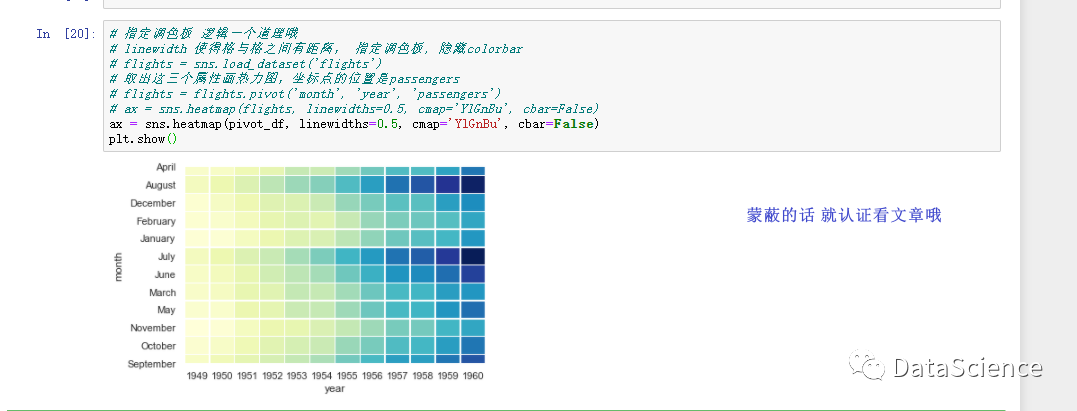## 雷达图

 # 数据准备
import numpy as np
import pandas as pd
import matplotlib.pyplot as plt
plt.rcParams['font.sans-serif'] = ['KaiTi']  # 显示中文字体•
items = np.array([u"品牌", u"价格", u"外观", u"硬件", u"拍照", u"电量"]) # u:unicode
scores= [88, 92, 70, 78, 79, 82]

# 画图数据准备，⻆度、score和angles的状态值
angles=np.linspace(0, 2*np.pi, len(items), endpoint=False)  #分割圆周长
scores=np.concatenate((scores,[scores]))
# concatenate:拼接，在原有的score和angles上加一
angles=np.concatenate((angles,[angles]))

# ⽤Matplotlib画蜘蛛图
fig = plt.figure() # 创建一个空白画布对象，添加子图可以将画板分成一行一列,形成一个绘图区
ax.plot(angles, scores, 'o-',linewidth=2) #连线
ax.fill(angles, scores, alpha=0.25) #上色
ax.set_thetagrids(angles * 180/np.pi, items) #写上标签
plt.show()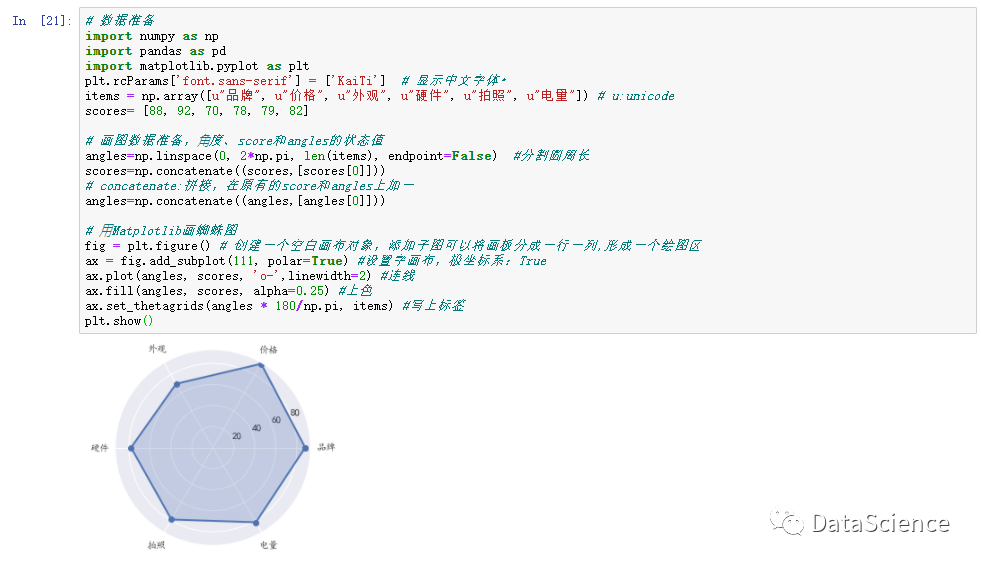## 二元变量分布

 # 数据准备
import seaborn as sns

# ⽤Seaborn画二元变量分布图（散点图，核密度图，Hexbin图）
sns.jointplot(x="total_bill", y="tip", data=tips, kind='scatter')
sns.jointplot(x="total_bill", y="tip", data=tips, kind='kde')
sns.jointplot(x="total_bill", y="tip", data=tips, kind='hex')
plt.show()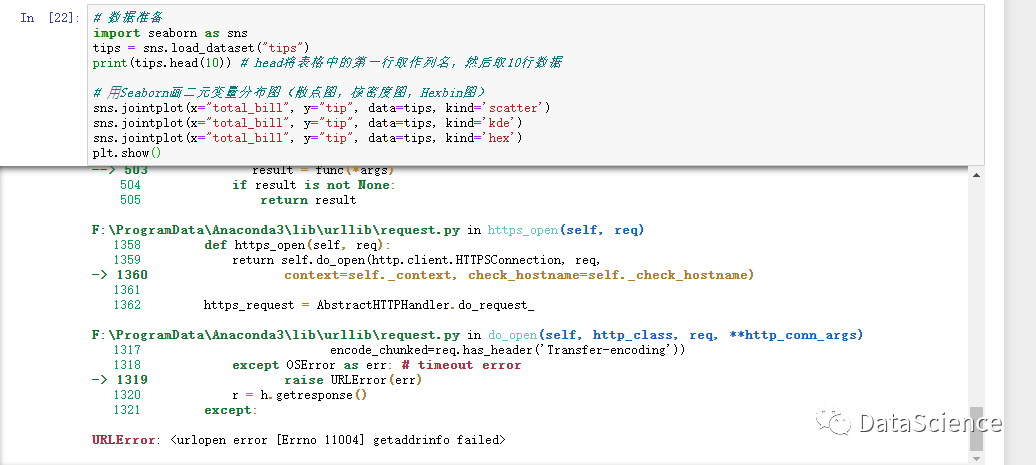### 引入数据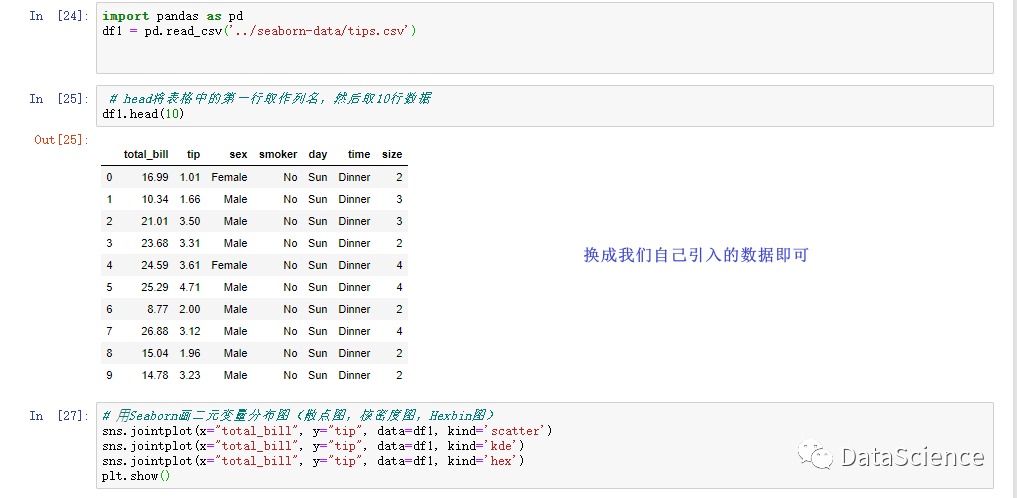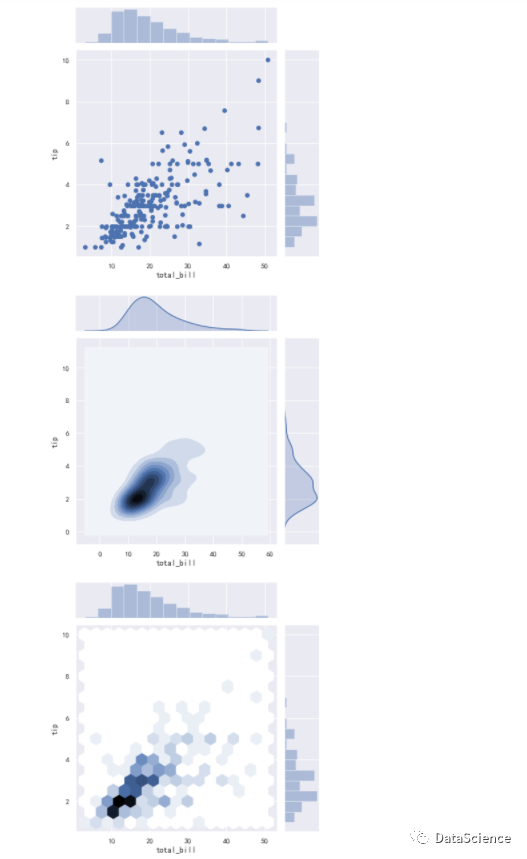## 成对关系

• Setosa

• Versicolour

• Virginica三个品种

• 在这个数据集中，针对每⼀个品种，都有50个数据，每个数据中包括了4个属性，分别是花萼长度、花萼宽度、花瓣长度和花瓣宽度。通过这些数据，可以预测出鸢尾花卉属于三个品种中的哪一种。

 # 数据准备
import seaborn as sns
# 用Seaborn画成对关系
sns.pairplot(iris)
plt.show()

⽤Seaborn中的pairplot函数来对数据集中的多个双变量的关系进行探索，如下图所示：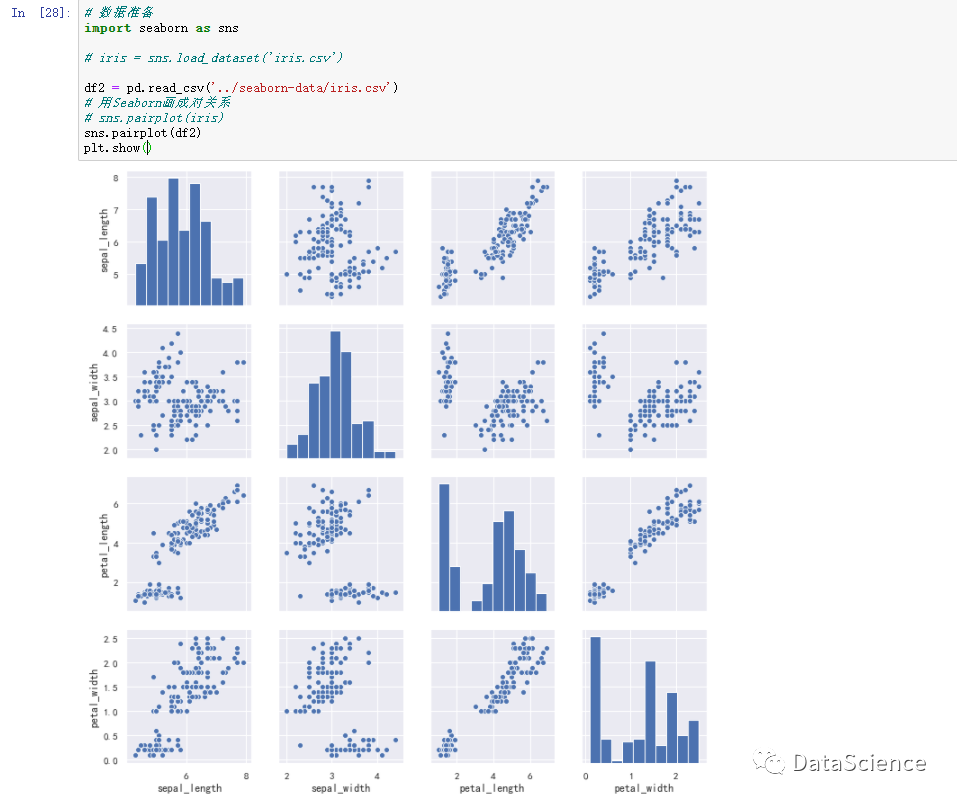## 小作业

• 1、Seaborn数据集中自带了car_crashes数据集，这是一个国外车祸的数据集，对这个数据集进行成对关系的探索。并用Seaborn画二元变量(x="total,y="speeding")分布图，如果想要画散点图，核密度图，Hexbin图该怎样写.

• 2、 探索更多seaborn-data 的数据展示

• 3、 你有应用的场景嘛？举个栗子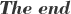-今日互动-

❤️来个「转发朋友圈」和「在看」，是最大的支持❤️

08-294171
05-04
09-244263
11-281249
04-188万+
04-033万+
06-10491
06-151万+
05-155944
12-305018
08-301157
11-221654
03-316万+
08-141万+
10-223万+
01-061万+
01-218554
07-022251
05-162万+

### “相关推荐”对你有帮助么？

•非常没帮助
•没帮助
•一般
•有帮助
•非常有帮助被折叠的  条评论 为什么被折叠?到【灌水乐园】发言「已注销」

¥2 ¥4 ¥6 ¥10 ¥20余额支付 (余额：-- )扫码支付获取中扫码支付点击重新获取扫码支付1.余额是钱包充值的虚拟货币，按照1:1的比例进行支付金额的抵扣。
2.余额无法直接购买下载，可以购买VIP、C币套餐、付费专栏及课程。余额充值